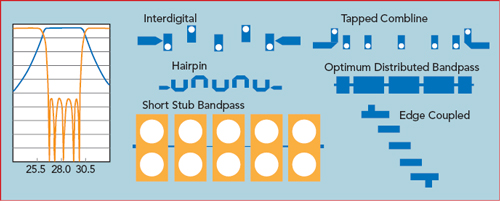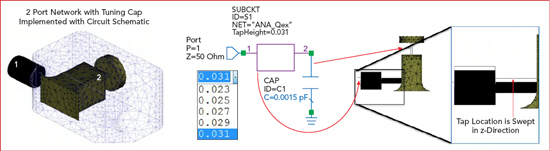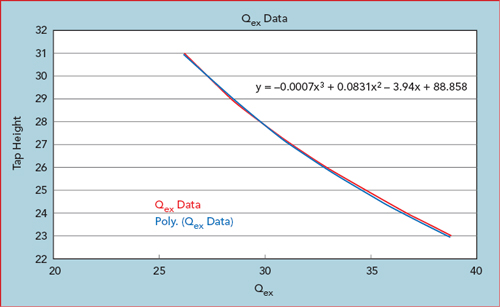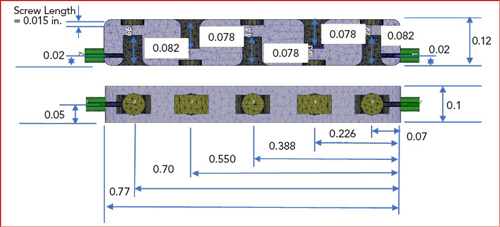www.microwavejournal.com/articles/32052-designing-a-narrowband-28-ghz-bandpass-filter-for-5g-applications

# Designing a Narrowband 28 GHz Bandpass Filter for 5G Applications

April 11, 2019

This article examines the factors driving the physical, electrical and cost constraints for 5G filters. To address these challenges, a narrowband filter design methodology using classic filter network theory, parameterized electromagnetic (EM) simulation and port-tuning techniques is presented. The approach is demonstrated using the NI AWR Design Environment platform to develop a narrowband 28 GHz bandpass cavity filter targeting mmWave backhaul applications.

5G will increase network capacity, reduce latency, and lower energy consumption through a number of innovative technologies aimed at enhancing spatial and spectral efficiency. The use of carrier aggregation, mmWave spectrum, base station densification, massive MIMO and beamforming antenna arrays will combine to support the goals of 5G communications at the cost of more signals operating in close spectral and spatial proximity. These enabling technologies place new demands on the filters required to mitigate signal interference across a dense network of base stations and mobile devices.

5G APPLICATIONS

5G will be deployed in stages to address three main thrusts; enhanced mobile broadband (EMBB), massive machine-type communication (mMTC) and ultra-reliable and low-latency communication (URLLC) for remote sensing and control for medical and autonomous vehicle applications. On the infrastructure side, densely-populated urban environments will utilize the mmWave frequency spectrum for higher data rates. Wireless backhaul is likely the most cost-effective and versatile solution to connect 5G base stations to the core network. Filters developed for the wireless backhaul application will face cost and volume challenges that must be considered early in the design stage.

DESIGN APPROACH AND FILTER SPECIFICATIONS

Ideal filter responses are well defined by math functions. This has led to the development of numerous commercial synthesis tools that can generate circuits for an exact filter response based on ideal element values; however, the parasitic behavior of the filter components must be considered early in the design stage. For this reason, synthesis is excellent for accelerating the initial design phase and generating mathematical filter solutions to serve as a starting point to define ideal lumped or distributed networks. Synthesis, however, is also limited in its ability to generate a physically-realizable filter. In this case, the synthesis tool provides critical coupling coefficients and external Q targets, but the ideal electrical design has limited usefulness.

Synthesis tools such as iFilter™ filter synthesis within NI AWR software can perform the math to produce ideal LC filters and precise distributed designs such as edge coupled, hairpin, interdigital and combline, based on ideal distributed microstrip and stripline models. Figure 1 shows several types of narrowband filters that can be synthesized based on microstrip technology with ideal distributed models that do not incorporate manufacturing limits and tolerances. Addressing these uncertainties can be very difficult without a process for converting ideal designs into physically-realizable ones.Figure 1 Several types of narrowband filters that can be synthesized based on microstrip technology.

The method used in this design is based on a technique introduced by Dishal and adopted for use with modern circuit and EM simulation by co-author Dan Swanson. EM modeling is used to efficiently determine three fundamental filter properties: the unloaded Q of the internal resonators, the coupling between two adjacent resonators and the external Q of the two resonators that form the input and output connections. Parametric studies with EM analysis are critical in modifying the physical structure in order to obtain specific values for these filter properties, which are determined by the Dishal method. Port tuning is then applied using circuit simulation and optimization with ideal lumped-element components, specifically, capacitors that are placed in strategic locations. Port tuning is used to guide adjustments that must be made to the final physical design.

Design by Optimization

General purpose optimizers are not particularly efficient for filter design unless they are able to take advantage of the mathematical foundation that defines a filter’s optimal response. For a lossless Chebyshev filter, the optimal behavior is an equal ripple insertion and return loss response in the pass band. Thus, if the optimizer can consistently find this equal ripple response, optimization can reliably be used. The optimizer that is used in this project is available as an add-on module to the NI AWR Design Environment platform using the software’s API COM interface to integrate fully with Microwave Office circuit design software and AXIEM and Analyst™ EM simulators.

Designing a Physically-Realizable 5G Filter

The design methodology follows a set of well-defined steps that scale for the desired frequency and bandwidth (see Figure 2). The process starts with specification of the filter requirements, including bandwidth, passband return loss and stopband rejection, from which the filter order is determined and the lowpass Chebyshev parameters are determined and scaled to the required frequency.Figure 2 Steps for designing a physically-realizable 5G filter.

An EM model of a single resonator is built, and its length for a desired resonant frequency and unloaded Q is determined. Additional EM models are created to generate the coupling coefficient and external Q curves that guide the determination of key physical dimensions such as resonator spacing and tap height. These individual components are then assembled, and port tuning is used to tweak the design for the optimum equal ripple response using optimization.

Narrowband Bandpass Filter Design

The filter is designed with a center frequency of 28 GHz, (3GPP band n257). The construction is based on a single in-line cavity using an interdigital arrangement to achieve 30 dB of rejection at 800 MHz off center frequency and an in-band return loss of 20 dB. This sets the in-band ripple at 0.044 dB. From these specifications, the expected insertion loss and the necessary filter order are determined.Figure 3 Coaxial resonator cross section.Figure 4 Coaxial resonator EM model.Figure 5 EM resonator simulation results for different resonator lengths.

The mathematical foundation for an ideal filter response is well established, with parameter values derived for an ideal lowpass Chebyshev filter response based on a cutoff frequency normalized to 1 Hz (see Table 1). Once the prototype ripple level is determined from the desired in-band return loss, the filter order N can be estimated based on the desired stopband rejection, as shown in Equation 1. A fifth-order filter is needed to achieve the desired selectivity and bandwidth.Where:

Rejection = Stopband Insertion Loss

RtnLoss = Passband Return Loss

S = Rejection Bandwidth/Filter Bandwidth

Design Details

The design is based on an interdigital configuration made up of coupled resonators with the open ends on a substrate or cavity alternately pointing in opposite directions. The length of the resonators determines the resonant frequency and the coupling between resonators is controlled by their separation. The width of the housing, for a cavity filter should be λ/4 at the operating frequency.

In addition to the cavity dimensions, another early concern is determining the cross-sectional dimensions of the resonator post. The resonator post cross section in relation to the outer cavity wall determines the characteristic impedance of the resonator. For a coaxial resonator, literature indicates an optimum resonator characteristic impedance of around 77 ohms, as determined by the resonator cross-section, resulting in a post-to-cavity width ratio of about 33 percent (see Figure 3). In this case, the optimum unloaded Q (Qu) is not achieved due to physical constraints. A coaxial transmission line calculation approximates the resonator Zo ~46 ohms.

Resonant Frequency and Qu Simulation

With the cavity and resonator cross-section dimensions determined, an EM model of a single resonator is defined with the resonator length parameterized so that the resonant frequency can be controlled, as shown in Figure 4. The EM model uses two coaxial feed structures that are loosely coupled to the waveguide cavity to act as the input and output ports. The frequency response for several different resonator lengths (see Figure 5), demonstrates that the resonant frequency increases with a shortening of the resonator.

The Qu of an individual resonator is calculated from the simulated time delay and insertion loss using Equation 2.For lowpass Chebyshev parameters, center frequency, and simulated Qu are shown in Table 2. An expected insertion loss of approximately 0.25 dB is calculated for the entire filter at mid-band using Equation 3.Where:

Δf is the equal ripple bandwidth of the filter

Qu is the expected average unloaded Q for the resonators

gi are the normalized lowpass filter element values, calculated for a given ripple in the Table 1.

An accurate accounting of manufacturing factors such as surface roughness and plating details are missing from the model used for EM simulation, so the in-band insertion loss will likely be higher than this initial estimate. The model approximates 80 percent of the ideal conductivity as a starting point. The quality of the silver plating is very process dependent. The measured data from the manufactured and tested filter can be used to adjust model conductivity information.

Coupling Coefficient Simulation

From the Chebyshev lowpass filter parameter values (g in Table 1) the external Q and the coupling coefficients (kij) for the resonant pairs are calculated based on Equations 3 and 4, respectively, using a 2.85 percent bandwidth.These calculated values provide the targets for the physical design. The next step is to build the coupling coefficient design curves using an EM model of the coupled resonators in order to determine the necessary spacing.Figure 6 Ports introduced for port tuning of coupled resonators.

Two resonators based on the initial resonator study are enclosed in a metal cavity and loosely coupled to the input and output ports, as shown in Figure 6. The resonators are identical and resonate at frequency f0. The coupling between the resonators results in a displacement Δf of the resonant frequencies, which is known as the coupling bandwidth. By dividing the coupling bandwidth by the ripple bandwidth of the filter, the normalized coupling coefficient is obtained. The normalized coupling coefficient divided by the center frequency provides the Chebyshev lowpass coupling coefficient. The resonant frequency occurs at the mid-point between the two peaks, as shown in Figure 7.Figure 7 Simulated transmission characteristics of two resonators enclosed in a metal cavity and coupled to input and output ports: cavity spacing equals 0.085 in. (a) and 0.125 in. (b).Figure 8 The f0 shift is addressed through tuning c1 and c2 values using optimization.

The more closely the resonators are spaced, the farther apart are the resonant peaks; this corresponds to higher coupling. As the resonators move farther apart the coupling gets progressively weaker and the two peaks merge together at the original resonant frequency. It can also be seen that the center frequency between the peaks in Figure 7a are shifted upward 74 MHz for the case where the resonator spacing is 85 mils. The admittance vs. frequency for these two ports is simulated and the capacitor values are optimized to zero out the admittance at 28 GHz, which re-centers the resonant frequency of the coupled pair (see Figure 8). The impact of the small amount of capacitance added or removed in order to center the coupled resonance can then be replaced by adjusting the resonator length to add/remove an equivalent amount of capacitance.

Calculating Kij Curves From Parametric EM Analysis

Dividing the normalized coupling coefficients by the 28 GHz center frequency provides the coupling coefficients that are needed to match up to the lowpass Chebyshev parameter values.

From Kij calculations:

[K1,2],[K4,5] = 0.02466

[K2,3],[K3,4] = 0.01812

Coupling bandwidth [1,2][4,5] =
690 MHz

Coupling bandwidth [2,3][3,4] =
507 MHz

(= Kij x 28GHz)Figure 9 Inverse relationship between the amount of coupling between resonators and their spacing.Figure 10 External coupling is found by measuring the 3 dB bandwidth of the resonance curve.

By parameterizing the spacing and tweaking the resonator length through port tuning, a curve relating coupling coefficients to very accurate resonator spacings based on EM analysis can be calculated. From this curve the spacing necessary to achieve a required coupling is determined. The curve in Figure 9 shows the anticipated inverse relationship between the amount of coupling between resonators and their spacing.

Parametric Modeling of the Tapped Resonator

The next step is to determine the physical details of the tapped resonators that provide the input/output to the filter. The external coupling is found by measuring the 3 dB bandwidth of the resonance curve denoted by Δf3dB (see Figure 10). The external Q is Qext = Qloaded = f0 / Δf3dB. It is also possible to determine the external Q by measuring the group delay of S11.

A parameterized EM model including a coax feed that taps into a single resonator is created and the distance from the bottom of the housing to the center of the coax tap is parameterized so that it can be adjusted to different heights to achieve the external Q calculated from the Chebyshev lowpass parameter. A lumped port is also placed between the resonator and tuning screw to support port tuning for addressing shifts in the resonator frequency due to the tap (see Figure 11).

EM analysis of the tapped resonator provides the time delay response as a function of frequency for different tap heights (see Figure 12). The time delay response is used to derive the external coupling. Parametric simulation enables the generation of an external Q vs. tap height curve, from which the tap height necessary for the required Qex can be directly chosen (see Figure 13).

Port Tuning

Parameterization is used to sweep values and generate the individual components that are combined to reproduce the entire filter and finalize the design through port tuning using the equal ripple optimization routine. While today’s EM simulators are quite fast and powerful, EM simulation times for low-order filters is still on the order of minutes or tens of minutes. Port tuning moves the optimization process from the EM domain to the circuit theory domain, where simulation times are much faster. Adding a port at each resonator enables rapid tuning of each resonator and the coupling between resonators (see Figure 14).Figure 11 Single resonator EM model includes a coaxial feed with a parameterized tap feed height to adjust external Q.Figure 12 Simulated reflected time delay for a given tap height.Figure 13 Qex vs. tap height based on a parameterized swept EM analysis of reflected time delay.Figure 14 Adding a port at each resonator enables tuning the frequency of each resonator and the coupling between resonators.

With each resonator loaded by a 50 ohm port, the raw coupling between resonators (not coupling coefficients per se) is simulated and the S-parameter variation across the simulation domain is extremely smooth (see Figure 15). In fact, for a narrowband filter, only five to 10 discrete frequencies across the simulation domain are required for the circuit simulator to generate a smooth frequency response plot through interpolation.Figure 15 Coupling parameter simulation (a) and resulting S-parameters (b).

With port tuning, the resulting capacitance values reveal the tuning requirements for the 3D EM model. Both positive and negative capacitance values can be used in circuit simulation. For the resonator tuning (port to ground capacitors), a negative capacitance value indicates that the resonator (EM model) is tuned too low. Positive capacitance represents a resonator that is tuned too high. For adjusting the coupling (port to port capacitors), a positive series capacitance indicates that the coupling is too strong in the EM model (the resonators are too close).

The process is repeated until the capacitances become sufficiently small. Convergence is guaranteed if the changes are not too large. Once resonator sensitivities (kHz per mm) are known, capacitance values can be converted into physical changes of the structure. Figure 16 shows the dimensions for the final design, derived from the port tuning.Figure 16 Final dimension of the filter design, derived from port tuning.

SIMULATED vs. MEASURED RESULTSFigure 17 Pre-plated cavity (a) and simulated vs. measured results (b).

From this design, the filter manufacturer (Reactel Inc.) built and tested the cavity filter, shown without the cover in Figure 17a. The frequency response of the measured filter and simulated model is shown in Figure 17b. As designed, the target response is achieved with moderate screw tuning. More precise tuning would better replicate the optimized, simulated result.

Manufacturing Tolerances and Yield Analysis

Modern CNC machines offer 0.0002 in. tolerances, not including tooling and fixturing. The relationship between 3D EM model and port tuning capacitors (resonators and coupling) can be used to perform yield analysis using the circuit simulator, allowing physical tolerances from manufacturing process to be translated into capacitor tolerances for yield analysis. Yield analysis of microwave circuits is often done with a Monte Carlo-type analysis with a large number of iterations. Running these iterations in the EM domain is prohibitive, but if the computed sensitivities convert a capacitance to a physical dimension, yield optimization is possible through the circuit simulation.

CONCLUSION

A practical design method that is independent of filter type/construction has been demonstrated, showing a robust equal ripple filter optimization that is a fast and intuitive alternative to design by synthesis and an efficient approach for port tuning complex EM-based filter models. EM tools continue to mature and add capabilities/speed, making it practical to include them in an optimization loop. This technique has been used to address the challenge of designing highly sensitive mmWave filter designs.